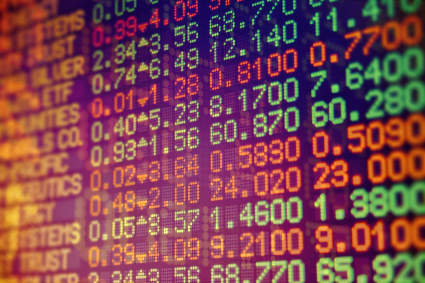# M-Score (Beneish)

The M-Score was developed and tested by Messod Beneish, an accounting professor at Indiana University in a 1999 paper “The Detection of Earnings Manipulation". The paper described a quantitative approach to detecting financial statement manipulation.

He based his model on forensic accounting principles, calling it the “probability of manipulation”, or “PROBM” or M-Score model.

He collected a sample of known earnings manipulating companies and identified their distinguishing characteristics and used those them to create a model for detecting manipulation. The model includes variables that are used to identify the effects of manipulation or reasons that may incentivize management to manipulate financial results.

When Prof Beneish tested the model he found that it identified approximately half of the companies involved in earnings manipulation prior to public discovery so it is a great quality metric to use.

### 12 out of 17 detected

The model, ahead of time, correctly identified 71% or 12 of the 17 highest-profile fraud cases in the period 1998 to 2002.

The Beneish M-score also proved useful in predicting returns.

In the 15-year period from 1993 to 2007 companies that were identified as potential earnings manipulators by the model returned 9.7% less each year compared to companies that were not identified.

Worth mentioning is that students from Cornell University using the M-score correctly identified Enron as an earnings manipulator, while experienced financial analysts failed to do so.

### How is it calculated?

The M score is based on a combination of the following eight different variables:

1. DSRI = Days’ Sales in Receivables Index
Measured as the ratio of days’ sales in accounts receivables in year t (this year) compared to year t-1 (previous year). A large increase in DSR could be indicative of sales manipulation.

2. GMI = Gross Margin Index
Measured as the ratio of gross margin in year t-1 to gross margin in year t.
If this variable is above 1 it means the gross margin has deteriorated. It assumes a company with bad prospects is more likely to manipulate earnings.

3. AQI = Asset Quality Index
Asset quality is measured as the ratio of non-current assets other than plant, property and equipment to total assets.
AQI is the ratio of asset quality in year t to year t-1.

4. SGI = Sales Growth Index
Ratio of sales in year t to sales in year t-1.
Sales growth is not itself a measure of manipulation, however, growth companies are likely to find themselves under pressure to manipulate sales to keep its “growth” image.

5. DEPI = Depreciation Index
Measured as the ratio of the rate of depreciation in year t-1 to the rate in year t.
DEPI greater than 1 indicates that assets are being depreciated at a slower rate. This suggests that the firm might be extending the useful life of its assets thus lowering depreciation and increasing profits.

6. SGAI = Sales, General and Administrative expenses Index
The ratio of SGA expenses in year t relative to year t -1.

7. LVGI = Leverage Index
The ratio of total debt to total assets in year t relative to yeat t-1.
An LVGI >1 indicates an increase in leverage

8. TATA - Total Accruals to Total Assets
Total accruals calculated as the change in working capital (excluding cash) minus depreciation.

The Beneish M Score Formula
The above eight variables are then weighted and added together as follows:
M = -4.84 + 0.92*DSRI + 0.528*GMI + 0.404*AQI + 0.892*SGI + 0.115*DEPI – 0.172*SGAI + 4.679*TATA – 0.327*LVGI

### Bigger than -2.22 is bad

A score greater than -2.22 indicates a strong likelihood of a company being a manipulator.

### How to use the ratio

Available as a screening ratio: No

Available as an output column ratio: Yes

### How to select the highest quality companies

To find companies with the best M-Score (Beneish) set a filter (click the funnel icon) and select all values smaller than -2.22.

If the M-Score = -999

If the M-Score is -999 it means that some values were missing and it could not be calculated.